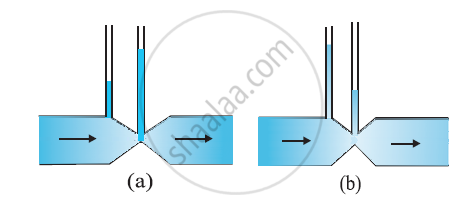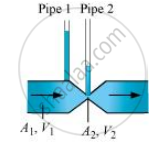CBSE (Science) Class 11CBSE
Share

# Figures (A) and (B) Refer to the Steady Flow of a (Non-viscous) Liquid. Which of the Two Figures is Incorrect? Why - CBSE (Science) Class 11 - Physics

ConceptBernoulli’S Principle

#### Question

Figures (a) and (b) refer to the steady flow of a (non-viscous) liquid. Which of the two figures is incorrect? Why?#### Solution 1

Figure (a) is incorrect. It is because of the fact that at the kink, the velocity of flow of liquid is large and hence using the Bernoulli’s theorem the pressure is less. As a result, the water should not rise higher in the tube where there is a kink (i.e., where the area of cross-section is small)

#### Solution 2

(a)

Take the case given in figure (b).Where,

A1 = Area of pipe1

A2 = Area of pipe 2

V1 = Speed of the fluid in pipe1

V2 = Speed of the fluid in pipe 2

From the law of continuity, we have:

A_1V_1 = A_2V_2

When the area of cross-section in the middle of the venturimeter is small, the speed of the flow of liquid through this part is more. According to Bernoulli’s principle, if speed is more, then pressure is less.

Pressure is directly proportional to height. Hence, the level of water in pipe 2 is less.

Therefore, figure (a) is not possible

Is there an error in this question or solution?

#### APPEARS IN

NCERT Solution for Physics Textbook for Class 11 (2018 to Current)
Chapter 10: Mechanical Properties of Fluids
Q: 15 | Page no. 269
Solution Figures (A) and (B) Refer to the Steady Flow of a (Non-viscous) Liquid. Which of the Two Figures is Incorrect? Why Concept: Bernoulli’S Principle.
S